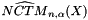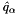#Boost C++ Libraries

...one of the most highly regarded and expertly designed C++ library projects in the world.

This is the documentation for an old version of boost. Click here for the latest Boost documentation.

## Struct template non_coherent_weighted_tail_mean_impl

boost::accumulators::impl::non_coherent_weighted_tail_mean_impl — Estimation of the (non-coherent) weighted tail mean based on order statistics (for both left and right tails)

## Synopsis

```// In header: <boost/accumulators/statistics_fwd.hpp>

template<typename Sample, typename Weight, typename LeftRight>
struct non_coherent_weighted_tail_mean_impl : public accumulator_base {
// construct/copy/destruct
non_coherent_weighted_tail_mean_impl(dont_care);

// public member functions
template<typename Args> result_type result(Args const &) const;
};```

## Description

An estimation of the non-coherent, weighted tail meanis given by the weighted mean of the

Equation 1.30.smallest samples (left tail) or the weighted mean of the

Equation 1.31.largest samples (right tail) above a quantileof level,being the total number of sample andthe sum of allweights:

Equation 1.32.Equation 1.33.### `non_coherent_weighted_tail_mean_impl` public construct/copy/destruct

1. `non_coherent_weighted_tail_mean_impl(dont_care);`

### `non_coherent_weighted_tail_mean_impl` public member functions

1. `template<typename Args> result_type result(Args const & args) const;`